# Methods and formulas for Generalized Variance Chart

Select the method or formula of your choice.

## Plotted points

|Si|, which is the determinant of the sample covariance matrix for sample i.

If no subgroups exist, all formulas for the Generalized Variance chart cannot be calculated. In this case, Minitab standardizes all values by subtracting the appropriate column mean and then dividing by the square root of the appropriate variance from the covariance matrix of all the data.

## Center line

|S|, the determinant of the sample covariance matrix.

## Control limits

### Lower control limit (LCL)

where:

The minimum LCL value is 0.

### Notation

TermDescription
p number of variables
n number of observations in each subgroup

## Methods and formulas for Box-Cox

### Box-Cox formula

If you use a Box-Cox transformation, Minitab transforms the original data values (Yi) according to the following formula:

where λ is the parameter for the transformation. Minitab then creates a control chart of the transformed data values (Wi). To learn how Minitab chooses the optimal value for λ, go to Methods and formulas for Box-Cox Transformation.

### Common λ values

The following table shows some commonly used λ values and their transformations.
λ Transformation
2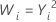0.5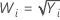0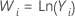−0.5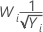−1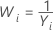1 D.C. Montgomery (2001). Introduction to Statistical Quality Control, 4th edition. John Wiley & Sons.
By using this site you agree to the use of cookies for analytics and personalized content.  Read our policy